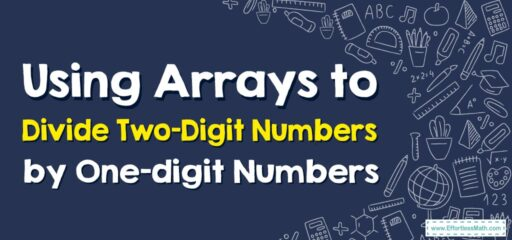# How to Use Arrays to Divide Two-Digit Numbers by One-digit Numbers

Using arrays can be a helpful way to visualize and understand the concept of division.## A Step-by-step Guide to Using Arrays to Divide Two-Digit Numbers by One-digit Numbers

Here’s a step-by-step guide for using arrays to divide a two-digit number by a one-digit number. We’ll use the example $$36÷6$$.

### Step 1: Draw an empty array

Draw an empty array with 6 squares in each row. The number of rows isn’t important right now, but make sure there are enough rows to reach 36 (the dividend).

### Step 2: Fill in the array

Begin filling in the squares with dots, or any symbol you like, until you reach the dividend (36 in this case). You should fill one row before moving on to the next.

### Step 3: Count the rows

Once you’ve filled in 36 squares, count the number of rows in your array. This represents the quotient (the answer to the division problem).

If you’ve done everything correctly, you should have 6 rows of 6 squares for a total of 36 squares. This shows you that $$36÷6=6$$.

Here’s what the array might look like:

⬛⬛⬛⬛⬛⬛
⬛⬛⬛⬛⬛⬛
⬛⬛⬛⬛⬛⬛
⬛⬛⬛⬛⬛⬛
⬛⬛⬛⬛⬛⬛
⬛⬛⬛⬛⬛⬛


This array shows that 36 divided by 6 equals 6 because there are 6 rows of 6 squares. The array helps to visualize the division process and reinforces the idea that division is the process of splitting a number into equal parts.

### What people say about "How to Use Arrays to Divide Two-Digit Numbers by One-digit Numbers - Effortless Math: We Help Students Learn to LOVE Mathematics"?

No one replied yet.

X
30% OFF

Limited time only!

Save Over 30%

SAVE $5 It was$16.99 now it is \$11.99